Courses

# Ex 2.2 NCERT Solutions - Polynomials Class 10 Notes | EduRev

## Class 10 : Ex 2.2 NCERT Solutions - Polynomials Class 10 Notes | EduRev

The document Ex 2.2 NCERT Solutions - Polynomials Class 10 Notes | EduRev is a part of the Class 10 Course Mathematics (Maths) Class 10.
All you need of Class 10 at this link: Class 10

Exercise 2.2
Ques 1: Find the zeroes of the following quadratic polynomials and verify the relationship between the zeroes and the coefficients.
(i) x2 - 2x - 8
(ii) 4s2 - 4s + 1
(iii) 6x2 - 3 - 7x
(iv) 4u2 + 8u
(v) t2 - 15
(vi) 3x2 - x - 4
Sol: (i) x2 - 2x - 8
We have p (x) = x2 - 2x - 8
= x2 + 2x - 4x - 8 = x (x + 2) - 4 (x + 2)
= (x - 4) (x + 2)
For p (x) = 0, we have
(x - 4) (x + 2) = 0
Either x - 4 = 0 ⇒ x = 4
or x + 2 = 0 ⇒ x = - 2
∴ The zeroes of x2 - 2x - 8 are 4 and - 2.
Now, sum of the zeroes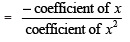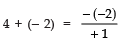2 = 2 [L.H.S. = R.H.S]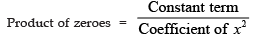4 × (- 2) = -8/1
⇒ - 8 = - 8 [L.H.S = R.H.S]
Thus, relationship between zeroes and the coefficients in x2 - 2x - 8 is verified.

(ii) 4s- 4s + 1
We have p (s) = 4s2 - 4s + 1
= 4s2 - 2s - 2s + 1 = 2s (2s - 1) - 1 (2s - 1)
= (2s - 1) (2s - 1)
For p (s) = 0, we have,
(2s - 1) = 0 ⇒ s = 1/2
∴ The zeroes of 4s2 - 4s + 1 are 1/2 and 1/2
Now,
Sum of the zeroes =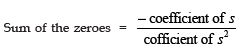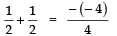⇒ 1 = 1 [L.H.S = R.H.S]
and Product of zeroes =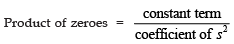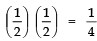⇒ 1/4 = 1/4  [L.H.S. = R.H.S]
Thus, the relationship between the zeroes and coefficients in the polynomial
4s2 - 4s + 1 is verified.

(iii) 6x2 - 3 - 7x
We have
p (x) = 6x2 - 3 - 7x
= 6x2 - 7x - 3
= 6x2 - 9x + 2x - 3
= 3x (2x - 3) + 1 (2x - 3)
= (3x + 1) (2x - 3)
For p (x) = 0, we have,
Either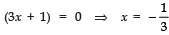or (2x - 3) = 0 ⇒ x =3/2
Thus, the zeroes of 6x- 3 - 7x are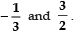Now,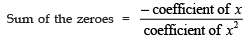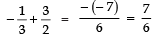⇒ and 7/6 = 7/6 [L.H.S = R.H.S]
and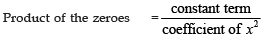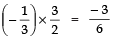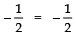[L.H.S = R.H.S]
Thus, the relationship between the zeroes and the coefficients in the polynomial
6x2 - 3 - 7x is verified.

(iv) 4u2 + 8u
We have, f(u) = 4u2 + 8u = 4u (u + 2)
For f (u) = 0,
Either 4u = 0 ⇒ u = 0
or u + 2 = 0 ⇒ u = - 2
∴ The zeroes of 4u2 + 8u are 0 and - 2.
Now, 4u2 + 8u can be written as 4u2 + 8u + 0.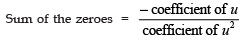⇒ 0 + (- 2) = -(8)/4
⇒ - 2 = - 2 [L.H.S. = R.H.S]
and the product of the zeroes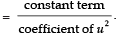⇒ 0 × (- 2) = 0/4
⇒ 0 = 0 [L.H.S. = R.H.S]
Thus, the relationship between the zeroes and the coefficients in the polynomial
4u2 + 8u is verified.
(v) t2 - 15
We have,
f (t) = t2 - 15 = (t)2 - (√15)2
(t+√15) = (t- √15)
For f (t) = 0, we have
Either ( t+√15) = 0 ⇒ t = -√15
or (t- √15) = 0 ⇒ t = √15
∴The zeroes of t2 - 15 are -√15 and √15.
Now, we can write t2 - 15 as t+ t - 15.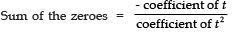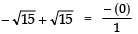⇒ 0 = 0 [L.H.S = R.H.S]
and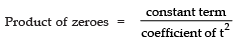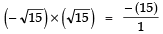⇒ − (15) = − (15) [L.H.S = R.H.S]
Thus, the relationship between the zeroes and the coefficients in the polynomial
t2 - 15 is verified.
(vi) 3x2 – x – 4
We have, f (x) = 3x2 - x - 4 = 3x+ 3x - 4x - 4
= 3x (x + 1) - 4 (x + 1)
= (x + 1) (3x - 4)
For f (x) = 0 ⇒ (x + 1) (3x - 4) = 0
Either (x + 1) = 0 ⇒ x = - 1
or 3x - 4 = 0 ⇒ x = 4/3
∴The zeroes of 3x- x - 4 are 1 and
Now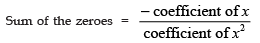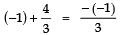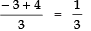⇒ 1/3 = 1/3    [L.H.S = R.H.S]
and Product of zeroes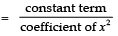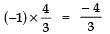⇒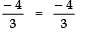[L.H.S. = R.H.S]
Thus, the relationship between the zeroes and the coefficients in 3x2 - x - 4 is verified.

Ques 2: Find a quadratic polynomial each with the given numbers as sum and product of its zeroes respectively: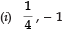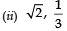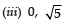(iv) 1, 1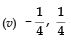(vi) 4, 1
Sol:

Note: A quadratic polynomial whose zeroes are α and β is given by
p (x) = {x2 -(α + β) x + αβ}
i.e., p (x) = {x2 - (sum of the zeroes) x + (product of the zeroes)}

(i) Since, sum of the zeroes, (α + β) =
Product of the zeroes, ab = - 1
∴ The required quadratic polynomial is
x2 - (α + β) x + ab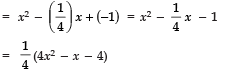Since,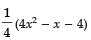and (4x2 - x - 4) have same zeroes, (4x2 - x - 4) is the required quadratic polynomial.

(ii) Since, sum of the zeroes, (α + β) = √2
Product of zeroes, αβ = 1/3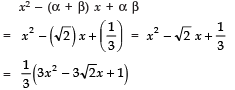Since,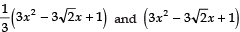have same zeroes,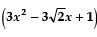is the required quadratic polynomial.

(iii) Since, sum of zeroes, (α + β) = 0
Product of zeroes, αβ = √5
∴ The required quadratic polynomial is
x2 - (α + β) x + αβ
= x2 - (0) x + √5
= x2 + √5

(iv) Since, sum of the zeroes, (α + β) = 1
Product of the zeroes = 1
∴ The required quadratic polynomial is
x2 - (α + β) x + αβ
= x2 - (1) x + 1
= x- x + 1

(v) Since, sum of zeroes,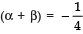Product of zeroes, αβ = 1/4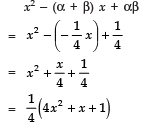Since, 1/4 (4x2 + x +1) and (4x2 + x +1) have same zeroes, (4x2 + x +1)the required quadratic polynomial is .

(vi) Since, sum of the zeroes, (α + β) = 4
Product of the zeroes, αβ = 1
∴ The required quadratic polynomial is
= x2 - (α + β) x + αβ
= x2 - (4) x + 1
= x2 - 4x + 1

• Division Algorithm for Polynomials
Let f (x) and g (x) be any two polynomials with g (x) = 0, then we can find polynomials q (x) and r (x) such that
f (x) = [g (x) × q (x)] + r (x)
where [degree of r (x)] < [degree of g (x)], where r (x) may also be zero.
This may be written as
Dividend = [Divisor × Quotient] + Remainder

Note: If α be a zero of the polynomial p (x), then (x − α) is a factor of p (x).

Offer running on EduRev: Apply code STAYHOME200 to get INR 200 off on our premium plan EduRev Infinity!

,

,

,

,

,

,

,

,

,

,

,

,

,

,

,

,

,

,

,

,

,

;